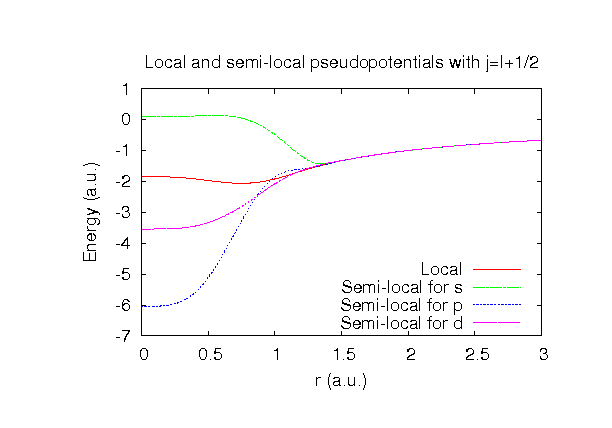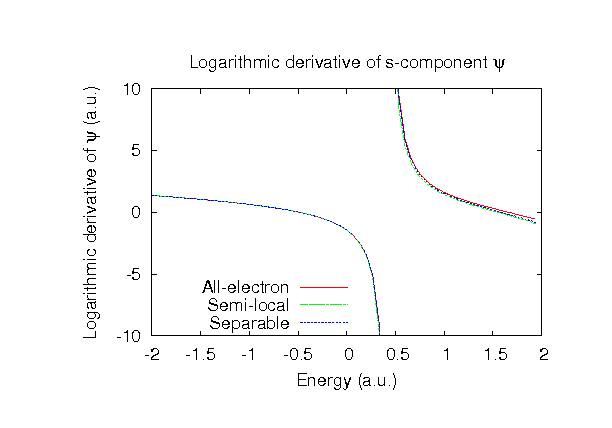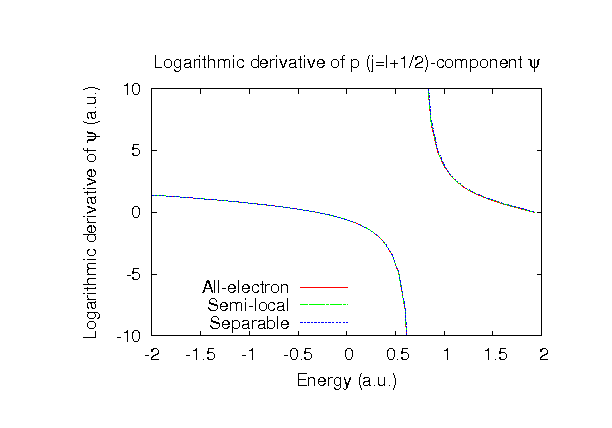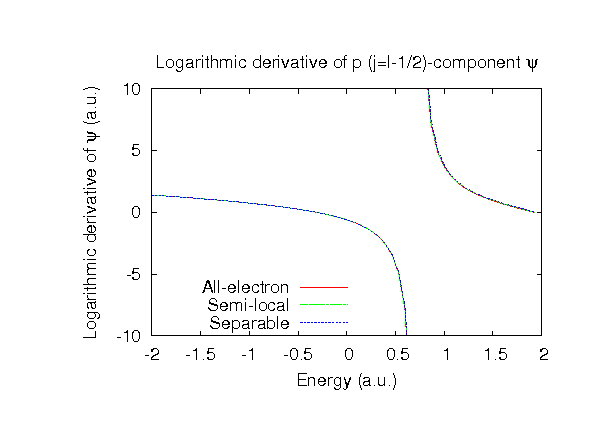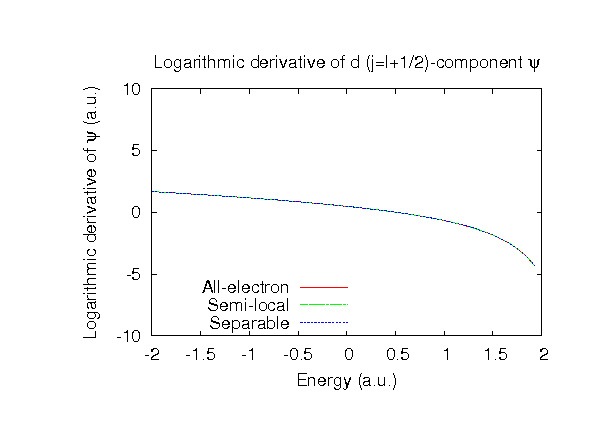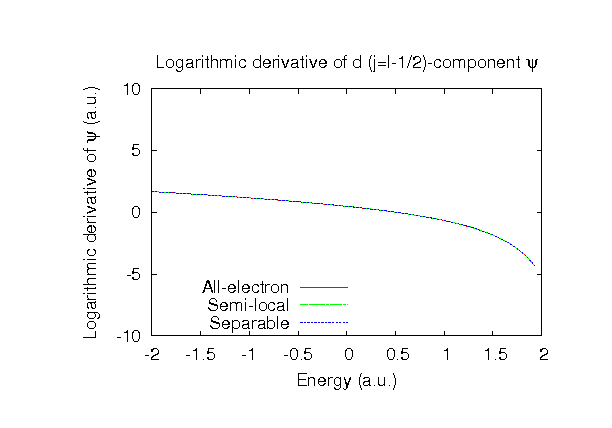### Fully relativistic pseudopotentials

Fully relativistic pseudopotentials generated by the MBK (PRB 47, 6728 (1993)) scheme within LDA (CA13) and GGA (PBE13) which contain a partial core correction and fully relativistic effects including spin-orbit coupling.

### Pseudo-atomic orbitals

The number below the symbol means a cutoff radius (a.u.) of the confinement potential. These file includes fifteen radial parts for each angular momentum quantum number l (=0,1,2,3). The basis functions were generated by variationally optimizing the corresponding primitive basis functions in the single atom and the FCC bulk. The input files used for the orbital optimization can be found at Be_opt.dat and Befcc_opt.dat . Since Be_CA13.vps and Be_PBE13.vps include the 2s state (2 electrons) as the valence states, the minimal basis set is Be*.*-s1. Our recommendation for the choice of cutoff radius of basis functions is that Be7.0.pao is enough for bulks, but Be8.0.pao or Be9.0.pao is preferable for molecular systems.

### Benchmark calculations by the PBE13 pseudopotential with the various basis functions

(1) Calculation of the total energy as a function of lattice constant in the fcc structure, where the total energy is plotted relative to the minimum energy for each case. a0 and B0 are the equilibrium lattice constant and bulk modulus obtained by fitting to the Murnaghan equation of state. The difference between Be7.0-s3p2 and Be7.0-s3p3d1 in the total energy at the minimum point is 0.025 eV/atom. An input file used for the OpenMX calculations can be found at Befcc-EvsV.dat . For comparison the result by the Wien2k code is also shown, where the calculation was performed by default setting in the Ver. 10.1 of Wien2k except for the use of RMT x KMAX of 12.(2) Calculations of the band dispersion in the fcc structure, where the non-spin polarized collinear calculation with the lattice constant of 3.20 Ang. was performed using Be_PBE13.vps, Be7.0-s3p2, and Be7.0-s3p3d1, and the origin of the energy is taken to be the Fermi level. The input file used for the OpenMX calculations can be found at Befcc-Band.dat . For comparison the result by the Wien2k code is also shown, where the calculation was performed by default setting in the Ver. 10.1 of Wien2k except for the use of RMT x KMAX of 12.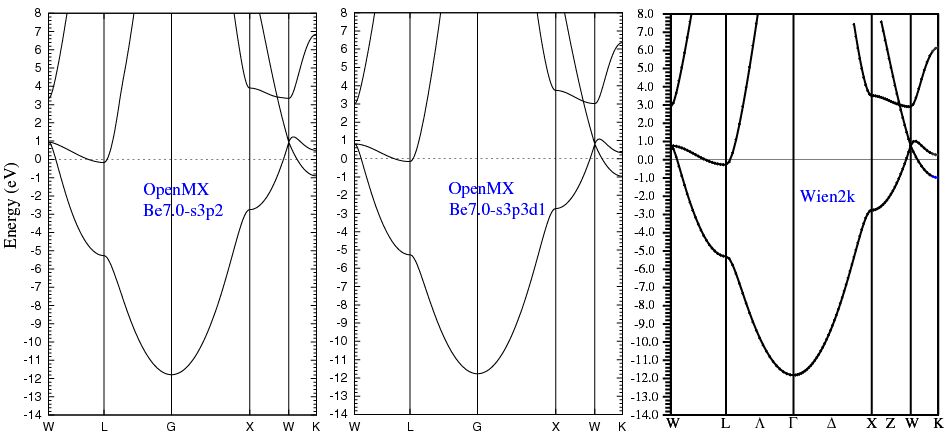(3) Calculations of a BeO molecule with S=1, where Be_PBE13.vps and Be9.0-s3p2 or Be9.0-s3p3d1 were used. The input files used for the OpenMX calculations can be found at BeO.dat , BeO_cp1.dat , BeO_cp2.dat , BeO_Be.dat , and BeO_O.dat .

 Equilibrium bond length (Ang.) Dipole moment (Debye) Atomization energy (eV) Atomization energy (couterpoise corrected) (eV) Be9.0-s3p2 1.368 5.57 4.90 4.89 Be9.0-s3p3d1 1.359 5.81 5.09 5.08 Other calc. 1.319 a 6.18 a 4.81 a Expt. 1.331 b - -

a B3LYP result: J.C. Pinheiro, F.E. Jorge, and E.V.R. De Castro, Int. J. Quantum Chem. 78, 15 (2000).
b K.P. Huber and G. Herzberg, Constants of Diatomic Molecules, Van Nostrand Reinhold, New York, 1979.

### Supplementary information for the GGA (PBE13) pseudopotential